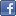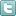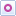EE Times-India > Power/Alternative Energy

Power/Alternative EnergyImplementing resonant mode using digital control

Posted: 20 Mar 2015Print Version

Keywords:LLC resonant power  AC-DC converter  Power Factor Correction  PFC  Pulse Width Modulation

The LLC resonant power stage has become an extremely popular power topology in the industry for high power applications that require an efficient power conversion up to and around a kilowatt. Resonant mode is a best fit choice as the second stage of the AC-DC converter as it is typically preceded by the boost Power Factor Correction (PFC) stage that provides a stable high voltage input.

In traditional Pulse Width Modulation (PWM) duty cycle controlled power converters the switching frequency is fixed while the duty cycle varies to maintain a regulated output voltage. In resonant power conversion the opposite occurs—duty cycle is fixed to 50% (minus deadtime) the frequency is allowed to vary.

Some of the advantages of the series resonant converter are:
• High efficiency
• ZVS/ZCS (Zero Voltage/current switching) of primary/secondary switches (depending upon operating mode)
• Increased power density
• Low noise and EMI due to ZCS
• No transformer saturation due to DC current block by resonant capacitor
• Good cross regulation between multiple outputs
• Flatter efficiency curve across the load range

However, there are some drawbacks that complicate the operation of the converter are:
• Soft start and light load operation
• Synchronous rectification drive
• Short circuit behaviour
• Difficult to design under a wide input operating range.

This article shows how the digital implementation of the resonant topology using an advanced digital controller (figure 1) makes the control, design, and optimisation an easy task and solves the common problems described above. The topology under consideration is the half bridge LLC resonant converter with MOSFETs as switches. However, the same concepts apply to the full bridge SRC (Series Resonant Converter) or even the LCC resonant topology.Figure 1: Simplified half bridge LLC power stage.

Resonant mode basics
Resonance can be defined as the excitation (or stimulus) that provides a maximum transfer of energy from one part (or element) of the system to another. In an ideal (lossless) system, this transfer of energy can continue indefinitely. Resonance occurs in several places in nature and such as electrical, mechanical, acoustical etc.

Figure 2 to figure 5 show an LCR circuit operating above and below resonance. It can be seen that only when the operating frequency is at resonance we can get maximum current flowing in the circuit. Another observation is when the excitation frequency (fS) is greater than the resonant frequency (fRES) the input current lags the input square voltage waveform which can be approximated by its first harmonic or fundamental by a sine. The reverse happens when fS < fRES , and the voltages and currents are in phase when fS= fRES. Due to the nature of the plots, we can conclude that when fS > fRES the voltage drops to zero before the current waveform then we can achieve ZVS turn-on of a switch (see figure 6). The opposite is true for fS < fRES.Figure 2: LCR resonant circuit.Figure 3: LCR resonant circuit excited at f= 95kHz< fRES. Red trace: Excitation voltage. Green trace: Current waveform.Figure 4: LCR resonant circuit excited at f= 97.95kHz= fRES. Red trace: Excitation voltage. Green trace: Current waveform.Figure 5: LCR resonant circuit excited at f= 100kHz> fRES. Red trace: Excitation voltage. Green trace: Current waveform.Figure 6: Primary side voltage and current waveforms showing ZVS turn on of a switch.

 Related Articles Editor's Choice
Comment on "Implementing resonant mode using dig..."

Top Ranked Articles

WebinarsVisit Asia Webinars to learn about the latest in technology and get practical design tips.

Search EE Times India
Services

Go to topConnect on FacebookFollow us on TwitterFollow us on Orkut
﻿